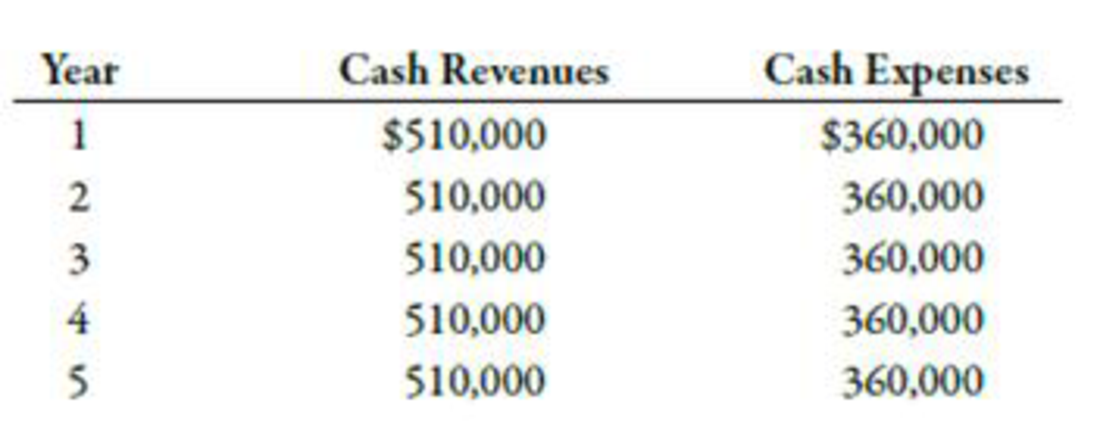Chapter 12, Problem 36E### Managerial Accounting: The Corners...

7th Edition
Maryanne M. Mowen + 2 others
ISBN: 9781337115773

#### Solutions

Chapter
Section### Managerial Accounting: The Corners...

7th Edition
Maryanne M. Mowen + 2 others
ISBN: 9781337115773
Textbook Problem
363 views

# Payback, Accounting Rate of Return, Net Present Value, Internal Rate of ReturnBlaylock Company wants to buy a numerically controlled (NC) machine to be used in producing specially machined parts for manufacturers of tractors. The outlay required is $384,000. The NC equipment will last 5 years with no expected salvage value. The expected after-tax cash flows associated with the project follow:Required:Compute the payback period for the NC equipment.Compute the NC equipment’s ARR. Round the percentage to one decimal place.Compute the investment’s NPV, assuming a required rate of return of 10%.Compute the investment’s IRR. 1. To determine Find out the payback period for NC equipment. Explanation Payback Period: The time taken by an investment to recover its original value is known as payback period. It is calculated by dividing the original amount of investment by annual cash flow from the investment. Use the following formula to calculate payback period: Paybackperiod=OriginalinvestmentAverageannualcashflow Substitute$384,000 for original investment and $150,0001 for average annual investment in the above formula. Paybackperiod=$384,000\$150,000=2

2.

To determine

Find out accounting rate of return (ARR) of NC equipment.

3.

To determine

Find out the NPV of the investment using required rate of return as 10%.

4.

To determine

Find out the IRR of the investment.

### Still sussing out bartleby?

Check out a sample textbook solution.

See a sample solution

#### The Solution to Your Study Problems

Bartleby provides explanations to thousands of textbook problems written by our experts, many with advanced degrees!

Get Started

#### Find more solutions based on key concepts